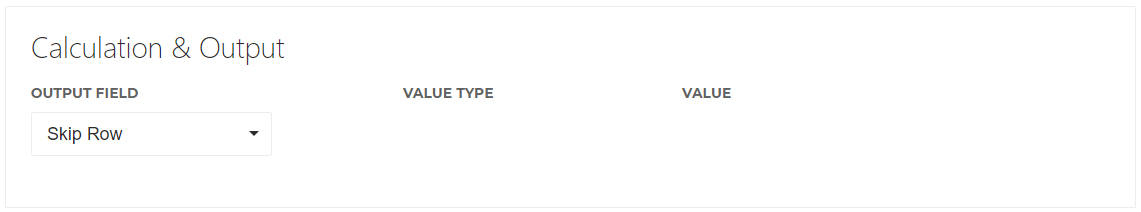# Calculations in a Template

Statements can contain all sorts of lines of data surplus to your reporting needs. “Totals” lines for units or revenue, or odd lines detailing balance transfers. In other instances the data may contain a Gross Amount & Commission but no Net Amount. Rules can be set to omit lines of data depending on certain conditions, run sums to generate required missing values, or concatenate data fields. Our goal is that with carefully programmed templates utilising appropriate necessary rules you should not need to make manual changes to your income files prior to uploading. These ‘rules’ are the Calculations.

There are two aspects to a Calculation. First, the Condition, specifies under which circumstance this calculation should happen. Second, the Calculation & Output, specify the calculation that should happen once the condition is met. As a bonus, at the bottom of your Calculation you have a Notes field where you can store information such as why you decided to create this calculation.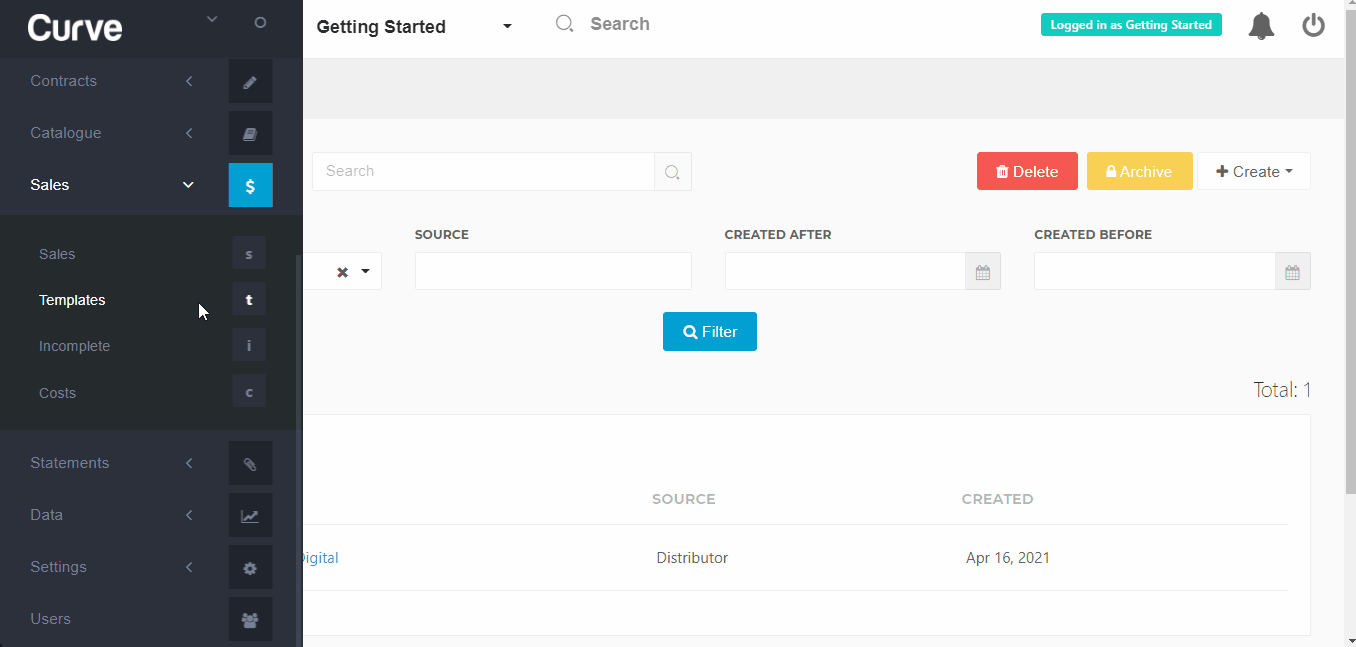Calculations are made in the order they appear on your Calculations tab. So contrary to your Contract Terms, the order of your calculations does make a difference.

# Conditions

Here you specify under which condition the following calculation should happen. You have three options.

Any One Of The Below – The calculation will happen when at least one condition in a list of conditions is met.
All Of The Below – The calculation will only happen when all of the conditions are met.
Always – The calculation will always happen. To put it in other words, there is no condition.

In the below example, we have selected “Any One Of The Below”, meaning our calculation will happen when either Column 1 or Column 2 of a particular sales line is empty. Had we selected “All Of The Below”, our calculation would only happen when both Column 1 & Column 2 were empty.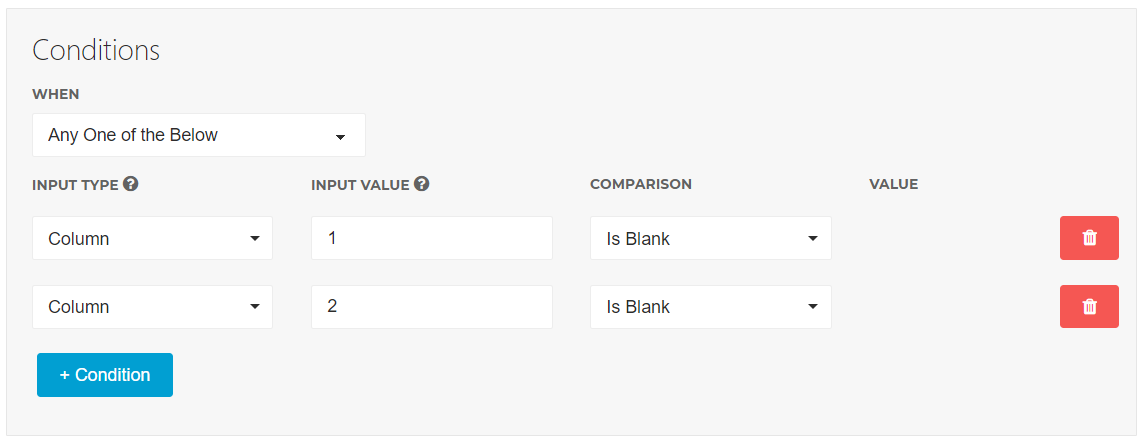# Calculation & Output

Calculations allow you to determine the value of a field by combining the data of a field/column/value with another field/column/value. It covers all basic mathematical calculations such as sums, differences, multiplications & divisions. Plus it has the ability to extract or concatenate characters from a value. When creating a new calculation, you will be presented the following fields:

Output Field – This decides the field in which the result of your calculation will be stored. One special option is the “Skip Row”, which allows you to skip an entire row entirely.
Value Type / Value – Here you decide which Values should be part of the Calculation. As input of the calculation you can pick either a column, field or value. “Column” takes the starting value from a column in the sales file. You then direct to which column in the subsequent Value table by entering a number, counting the columns in the data from left to right. “Field” takes the starting value from a field matched in the income file. So when selecting this, the subsequent Value box is populated with the various Field names that are matched to columns on the template overview tab. Make sure the directed field actually contains some data & is linked to a column when setting up the template. With “Value” as a Value Type, you enter an absolute number that will be used in the calculation.
Calculation – This decides which calculation should be made between the chosen Values. You can select Plus, Minus, Multiplied By, Divided By, Get First X Numbers Of Characters, Get Last X Numbers Of Characters, or combine the the data via a Concatenate function.

Let’s look at some examples of how to create calculation outputs.

#### Plus/Minus/Multiply/Divide

This example calculates & stores the Net Amount value by multiplying the mapped Gross Amount field by 0.9. It performs this calculation on every individual sales line in the statement. Essentially this is applying a 10% commission. For this to work the Gross Amount field would need to be set to a Column in the Template.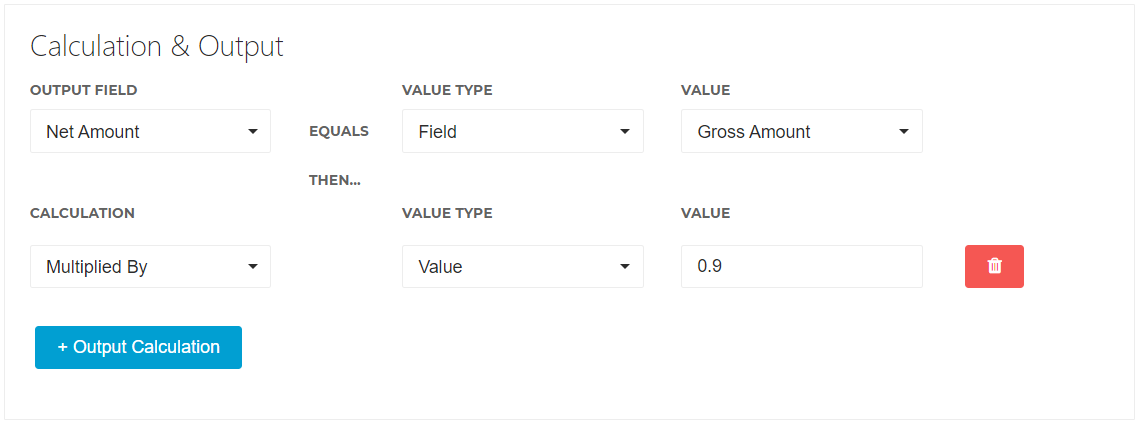Additions, subtractions & divisions are done in the same way. It is possible to perform a series of calculations by adding a new calculation layer; just hit the  + Output Calculation button. The system will make the calculations in the order they are added.

#### Get X Numbers of Characters

This function trims the data to the first or last specified number of characters. The following example will take the first 10 characters of Column 5 & store it as the Barcode. if Column 5 holds the value  7856957595 GTIN, Curve will store 7856957595 as the Barcode of your sales line.

####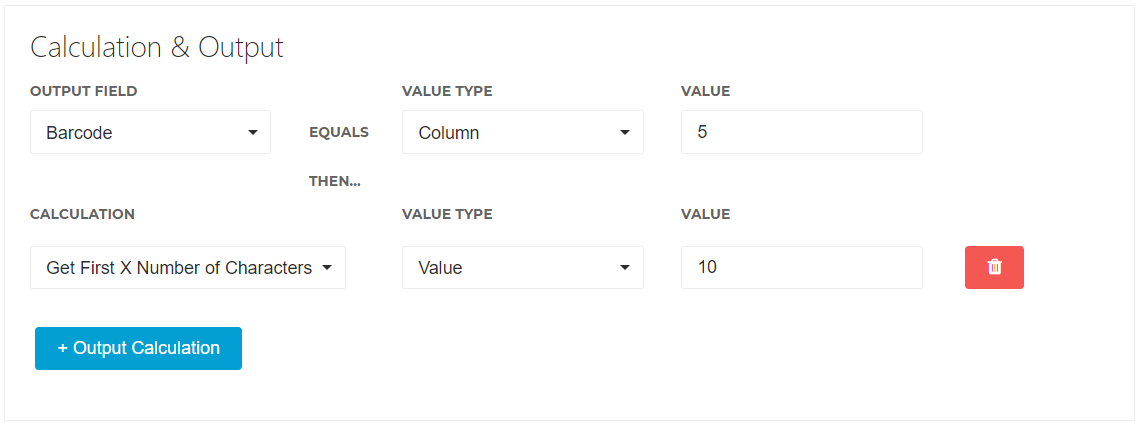Concatenate

The Concatenate function combines data from different columns into one. This is typically used to combine text data. The following example combines the data in Column 5 & Column 6 to store it as the Original Configuration.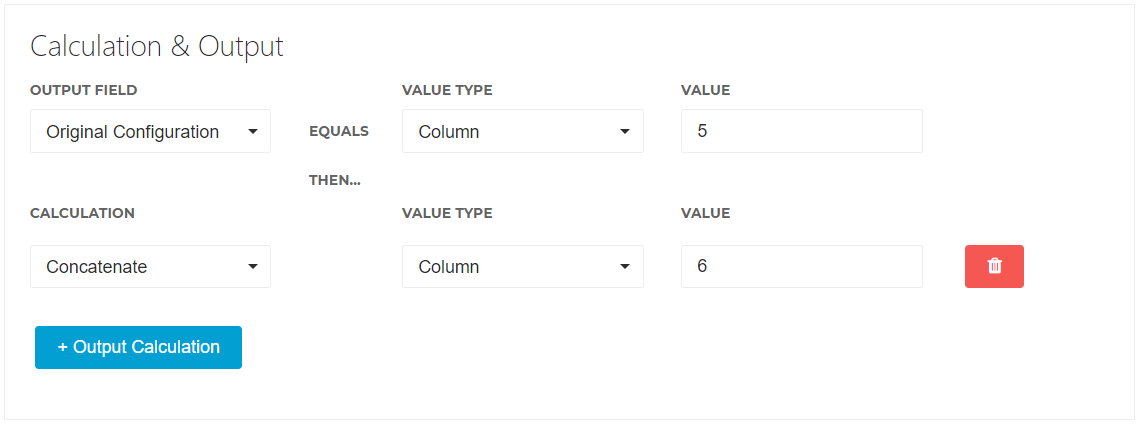If a sales line holds the value Online in Column 5 and the value Stream in Column 6, then Curve will store as the Original Configuration the value OnlineStream. You will then be able to match this combination of data to your preferred Configuration in your Settings.

#### Skip Row

The Skip Row functions allows you to tell Curve to ignore certain rows of data under specific conditions. A common use case scenario for this is a Total row at the bottom of your statement you wish to ignore. Or perhaps you prefer to leave out certain royalty lines with a 0 Net Amount.

First, via the Conditions area, you will need to specify under which circumstances you wish Curve to ignore the sales line. In the Output Field, you then select the value Skip Row.